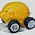## Saturday, May 2, 2015

### Equilibrium in the economic potential picture

It might have been a bit confusing to see $N$ on both sides of the second equation in this post. That's because I used an equilibrium relationship to solve for the $T S$ term. If you use an equilibrium relationship for all three terms you actually come out with all the terms being proportional to $N$. Let's see how that works ...

$$N \approx c N/\kappa + \kappa P M + \alpha p X$$

For the second term, we have

$$\kappa P M = \kappa \frac{P_{0}}{\kappa} \left( \frac{M}{M_{0}} \right)^{1/\kappa - 1} M$$
$$= \kappa \frac{P_{0}}{\kappa} \left( \frac{M}{M_{0}} \right)^{1/\kappa - 1} M_{0} \frac{M}{M_{0}}$$
$$= P_{0} M_{0} \left( \frac{M}{M_{0}} \right)^{1/\kappa}$$
$$= P_{0} M_{0} \frac{N}{N_{0}} \equiv \eta N$$

And for the third term, assuming the market $p : N \rightarrow X$ with information transfer index $k$, we have

$$\alpha p X = (\alpha/k) N \equiv \gamma N$$

Putting this all together, we have:

$$N \approx c N/\kappa + \eta N + \gamma N$$

$$= ( c/\kappa + \eta + \gamma ) N$$

or

$$c/\kappa + \eta + \gamma \approx 1$$

with each constant term representing the fractional contribution of each piece of the economy; in this case: entropy (i.e. macro gains from having a large diverse economy), money and goods.

1.I like how your vocabulary is evolving...macro gains...unfortunately this seems meaningless...is it good to have a large entropy part or bad?

1.Hi LAL,

That is a really good question.

To some degree, it's neither good nor bad -- it exists in any economy.

However the coefficient is c/kappa, and since all of the parameters for the different countries I've looked at are about the same (they all fall along that dashed path in the graph in the upper right of this blog's website viewed on a desktop), the biggest contributor to differences would be kappa -- which ranges from 0.4 to 1.1 in the cases I've looked at.

If kappa is 0.4, then there is a large T*S component (relative to other countries). A kappa of 0.4 also means there is high inflation, high NGDP growth and the quantity theory of money is a good approximation.

If kappa is 1.1, then there is a smaller T*S component and a low inflation/low growth/liquidity trap economy.

From that perspective, it seems the former is the better situation -- assuming a competent central bank able to smooth out the business cycles.

However a larger entropy component would make your economy more vulnerable to coordination problems (i.e. panics and other market fluctuations that coordinate pessimistic behavior) ... a smaller entropy component means market herding behavior has less of an impact.

Those are at least my initial thoughts ...

2.This comment has been removed by the author.